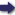(London :  Kegan Paul, Trench, Trübner & Co.,  1910.)

 Tools

## Search this bookPrev Page 275 Next``` CHAPTER XXVI. 275 H T, i.e. 3431'. So we get as quotient T C = 0° 14' 45''; and B C, the stature of the observer, is 0° 7' 45'^ Our calculation is based on this, that H B, the sinus totus, is 3438^ However, the radius of the earth is, according to the circumference which we have men¬ tioned, 795° 27' 16'' (yojana). If we measure B C by this measure, it is = I yojana, 6 la^osa, 1035 yards ( = 57,035 yards). If we suppose B C to be equal to four yards, it stands in the same relation to A T, according to the measure of the sine, as 57,035, i.e. the yards which we have found as the measure of the stature, to A T according to the measure of the sine, i.e. 22\$. If we now calculate the sine, we find it to be 0° o^ i" '^"', and its arc has the same measure. However, each degree of the rotundity of the earth represents the measure of 13 yojana, 7 krosa, and 333 j yards (sic). Therefore the field of vision on the earth is 291| yards (sic). Page 138. (Por an explanation of this calculation see the notes.) The source of this calculation of Balabhadra's is the Pulisasiddhdnta, which divides the arc of the quarter of a circle into 24 kardajdt. He says : " If anybody asks for the reason of this, he must know that each of these kardajdt is yig- of the circle = 225 minutes ( = 3|- degrees). And if we reckon its sine, we find it also to be = 225 minutes," This shows us that the sines are equal to their arcs in parts which are smaller than this kardaja. And because the sinus totus, according to Pulisa and Aryabhata, has the relation of the diameter to the circle of 360 degrees, this arithmetical equality brought Balabhadra to think that the arc was perpen¬ dicular ; and any expanse in which no convexity pro¬ trudes preventing the vision from passing, and which is not too small to be seen, is visible. This, however, is a gross mistake; for the arc is never perpendicular, and the sine, however small it be, never equals the arc. This is admissible only for such degrees as are supposed for the convenience of ```Prev Page 275 Next